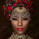24314 views
24314
Here's my version of the MACD _BB . This is a great indicator to capture short term trends.

yellow candles = long
aqua candles = short

This indicator can be much better. I will work on it and publish an improved version (hopefully) soon. In the mean time , go ahead and play around with the code, and please share your findings :)

Cheers

Algo
//AK MACD BB
//created by Algokid , February 24,2015

study("AK MACD BB v 1.00")

length = input(10, minval=1, title="BB Periods")
dev = input(1, minval=0.0001, title="Deviations")

//MACD
fastLength = input(12, minval=1)
slowLength=input(26,minval=1)
signalLength=input(9,minval=1)
fastMA = ema(close, fastLength)
slowMA = ema(close, slowLength)
macd = fastMA - slowMA

//BollingerBands

Std = stdev(macd, length)
Upper = (Std * dev + (sma(macd, length)))
Lower = ((sma(macd, length)) - (Std * dev))

Band1 = plot(Upper, color=gray, style=line, linewidth=2,title="Upper Band")
Band2 = plot(Lower, color=gray, style=line, linewidth=2,title="lower Band")
fill(Band1, Band2, color=blue, transp=75,title="Fill")

mc = macd >= Upper ? lime:red

// Indicator

plot(macd, color=mc, style =circles,linewidth = 3)
zeroline = 0
plot(zeroline,color= orange,linewidth= 2,title="Zeroline")